﻿ PSdropout卷积神经网络在危化品巡检车中的应用
«上一篇文章快速检索 高级检索

 智能系统学报2020, Vol. 15Issue (6): 1131-1139  DOI: 10.11992/tis.2020070220

### 引用本文SHA Yun, LI Qifei, GAN Jianwang, et al. Application of PSdropout convolutional neural network in inspection car for hazardous chemicals[J]. CAAI Transactions on Intelligent Systems, 2020, 15(6): 1131-1139. DOI: 10.11992/tis.202007022.### 文章历史

PSdropout卷积神经网络在危化品巡检车中的应用

Application of PSdropout convolutional neural network in inspection car for hazardous chemicals
SHA Yun, LI Qifei , GAN Jianwang , LIU Xuejun , WEI Li’ang
School of Information Engineering, Beijing Institute of Petrochemical Technology, Beijing 102617, China
Abstract: The storage environment of hazardous chemicals is complex and changeable. To adapt to different environments, visual-inspection vehicles based on convolutional neural networks require fast training methods, but the problem of improving the training speed of convolutional neural networks remains to be solved. To improve the training speed of the algorithm, effective neural cell in network must be extracted from the network more quickly. In the traditional algorithm, the removal of neurons from the fully connected layer is typically performed using the dropout method, which is based on the Bernoulli distribution hypothesis. In this paper, we propose a dropout method based on the Poisson distribution. Theoretically, by making full use of the historical behavior of neurons, the maximum likelihood function based on the Poisson distribution is similar to that based on the Bernoulli distribution. The experimental results show that while maintaining the correct rate, the training can be converged in advance, thereby saving the training time.
Key words: storage of hazardous chemicals    inspection vehicle    convolutional neural network    dropout    Poisson distribution    subnetwork    full link layer    network architecture

1 相关工作 1.1 卷积神经网络

1.2 具体实现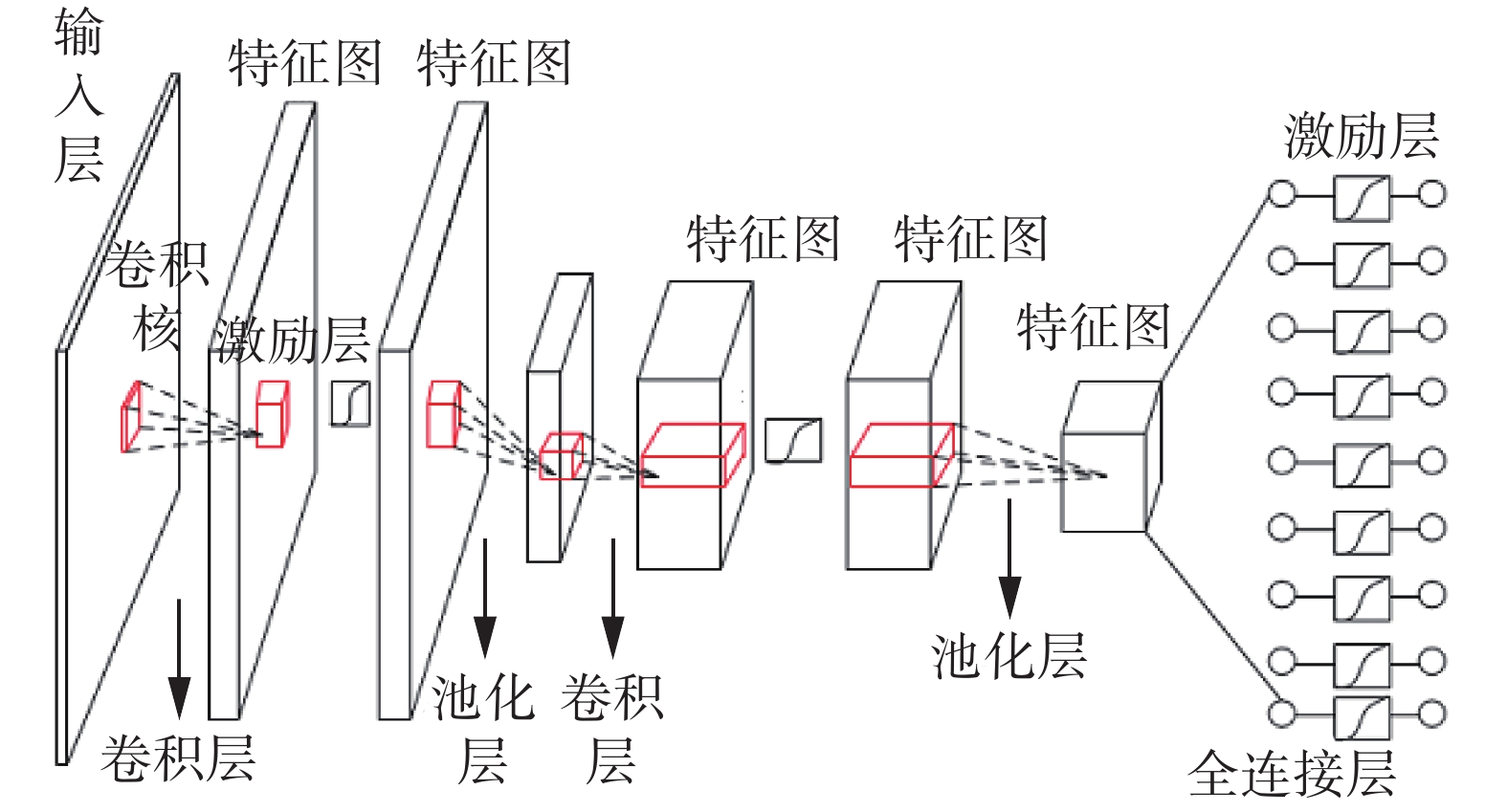Download: 图 1 网络架构 Fig. 1 Network architecture

 $L\left( {\theta ,{\theta ^*},X} \right) = {\rm{MSE}} = \frac{1}{n}{\sum\limits_{i = 1}^n {\left\| {\widetilde {{y_i}}\left( {X,\theta _i^*} \right) - {y_i}\left( {X,{\theta _i}} \right)} \right\|} ^2}$

2 PSdropout抽样算法

2.1 理论分析及算法步骤

 $\begin{array}{c} z_i^{\left( {l + 1} \right)} = w_i^{l + 1}{y^l} + b_i^{l + 1}\\ y_i^{\left( {l + 1} \right)} = f\left( {z_i^{l + 1}} \right) \end{array}$

2012年，Hinton在论文中提出Dropout，当一个复杂的神经网络用于训练特征较为简单的数据集时，会很容易出现过拟合现象，主要表现为训练时测试准确率很高、测试时测试准确率很低。为了防止过拟合，可以通过阻止特征检测器的共同作用来提高神经网络的性能。

 $r_j^{\left( l \right)} \sim B\left( {{p_m}} \right)$ (1)
 $\mathop {{y^l}}\limits^ \sim = {r^{\left( l \right)}}*{y^{\left( l \right)}}$ (2)
 $z_i^{\left( {l + 1} \right)} = w_i^{\left( {l + 1} \right)}\mathop {{y^l}}\limits^ \sim + b_i^{\left( {l + 1} \right)}$ (3)
 $y_i^{\left( {l + 1} \right)} = f\left( {z_i^{\left( {l + 1} \right)}} \right)$ (4)

Dropout方法神经元去留概率服从伯努利分布，根据式(1)~(4)对网络框架进行更新。DropConnect不再是随机将隐含层节点的输出清0,而是将节点中的每个与其相连的输入权值以一定的概率清0，在输入处修剪掉多余的连接，在Dropout概率设置为0.5时在本层网络中可以节省一半的计算量。以此算法作为实验中的对比算法。

 ${p_m} = \frac{{{C_w}}}{{{R_{{\rm{times}}}}}}$ (5)
 $p_m^\prime = \left\{ \begin{array}{l} 1,\;\;{p_m} \geqslant {p_0} \\ 0,\;\;{p_m} < {p_0} \end{array} \right.$ (6)
 $r_j^{(l)} \sim P\left( {p_m^\prime } \right)$ (7)
 $\tilde {{y^l}} = r_j^{\left( l \right)}*{y^{\left( l \right)}}$ (8)
 $z_i^{\left( {l + 1} \right)} = w_i^{\left( {l + 1} \right)}\tilde {{y^l}} + b_i^{\left( {l + 1} \right)}$ (9)
 $y_i^{\left( {l + 1} \right)} = f\left( {z_i^{\left( {l + 1} \right)}} \right)$ (10)

2.2 理论推导

Dropout与PSdropout抽样算法不同之处在于神经元的去留服从分布不同，极大似然估计的依据是概率最大的事件最可能发生，在神经元去留问题上，神经元激活不同数目可作为不同事件，改进的PSdropout使具有积极意义的神经元最大化的激活这一事件发生概率可由极大似然估计进行推导。另外，在理论中找相等是很难的，泊松分布和伯努利分布的极大似然相似，即可证明其有一定的相似性。对比伯努利分布与泊松分布，观测二者的极大似然估计值如下：

Dropout：在实验中，神经元去留概率服从伯努力分布：

 $D = \{ y_1,y_2, \cdots ,y_n\},\;\;y \sim B(\mu )$

 $L = p\left( {D\left| \mu \right.} \right) = \displaystyle\mathop \Pi \limits_{n = 1}^N p\left( {{y_n}\left| \mu \right.} \right) =\displaystyle \mathop \Pi \limits_{n = 1}^N {\mu ^{{y_n}}}{\left( {1 - \mu } \right)^{\left( {1 - {y_n}} \right)}}$

 $\begin{array}{c}l = \ln p\left( {D\left| \mu \right.} \right) = \displaystyle\sum\limits_{n = 1}^N {\ln \left\{ {{\mu ^{{y_n}}}{{\left( {1 - \mu } \right)}^{\left( {1 - {y_n}} \right)}}} \right\}} = \\ \displaystyle\sum\limits_{n = 1}^N {\left\{ {{y_n}\ln \mu {\rm{ + }}\left( {1 - {y_n}} \right)\ln \left( {1 - \mu } \right)} \right\}}\end{array}$

 $\begin{array}{*{20}{c}} {\dfrac{{{\rm{\partial}} l}}{{\partial \mu }} = \displaystyle\sum\limits_{n = 1}^N {\left\{ {{y_n}\dfrac{1}{\mu }\left( {1 - {y_n}} \right)\dfrac{1}{{1 - \mu }}} \right\}} = \dfrac{1}{{\mu \left( {1 - \mu } \right)}}\left\{ {{y_n} - \mu } \right\} = 0}\\ {{\mu _{{\rm{MLE}}}} = \dfrac{1}{N}\displaystyle\sum\limits_{n = 1}^N {{y_n}} } \end{array}$

PSdropout抽样算法：在实验中，神经元的去留服从泊松分布：

 $D = \{ y_1,y_2, \cdots ,y_n\},\; y \sim P(\lambda )$

 $L = \mathop \Pi \limits_{n = 1}^N \frac{{{\lambda ^{{y_n}}}}}{{{y_n}!}}{{\rm{e}}^{ - \lambda }} = {{\rm{e}}^{ - n\lambda }}\mathop \Pi \limits_{n = 1}^N \frac{{{\lambda ^{{y_n}}}}}{{{y_n}!}}$

 $l = \ln L = - n\lambda + \sum\limits_{n = 1}^N {\left( {{y_n}\ln \lambda - \ln {y_n}} \right)}$

 $\begin{array}{*{20}{c}} {\dfrac{{\partial l}}{{\partial \lambda }} = - N + \displaystyle\sum\limits_{n = 1}^N {\dfrac{{{y_n}}}{\lambda }} = 0}\\ {{\lambda _{{\rm{MLE}}}} = \dfrac{1}{N}\displaystyle\sum\limits_{n = 1}^N {{y_n}} } \end{array}$

3 实验

3.1 数据集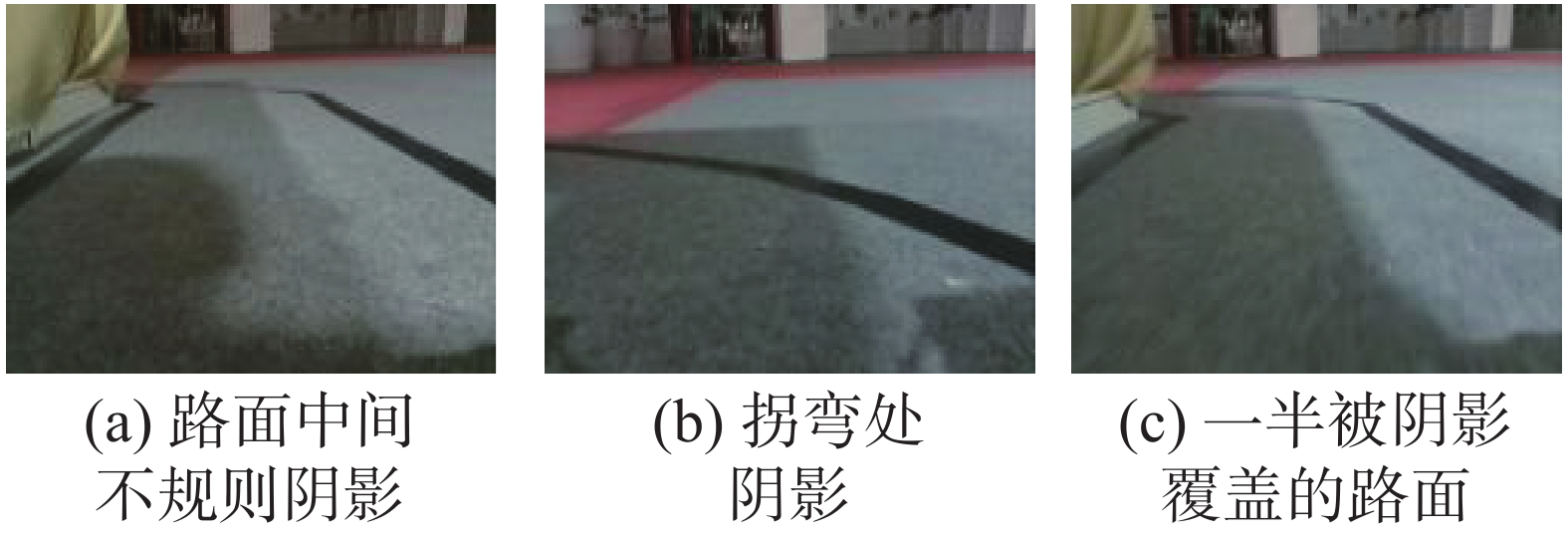Download: 图 2 原始场景样本图 Fig. 2 Sample pictures of original scene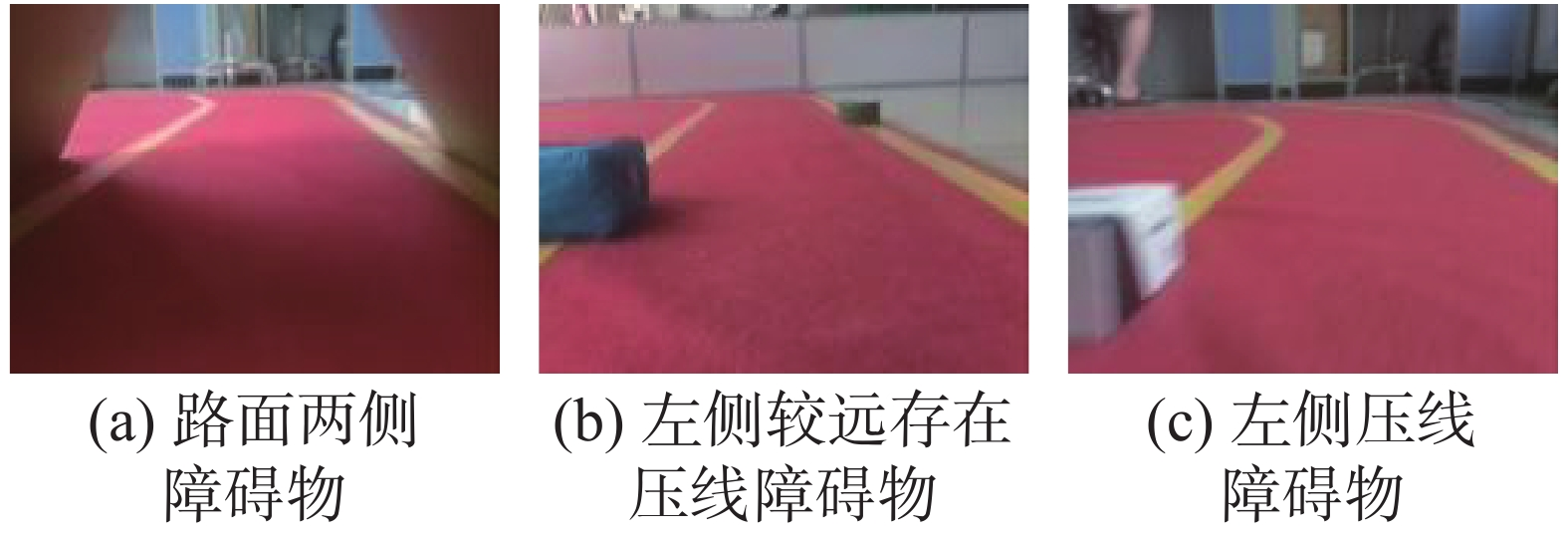Download: 图 3 复杂场景样本图 Fig. 3 Sample photos of complex scene
3.2 单场景数据量测试实验表 1 loss统计结果 Tab.1 Statistical loss results表 2 测试结果 Tab.2 Statistical test results

3.3 多场景测试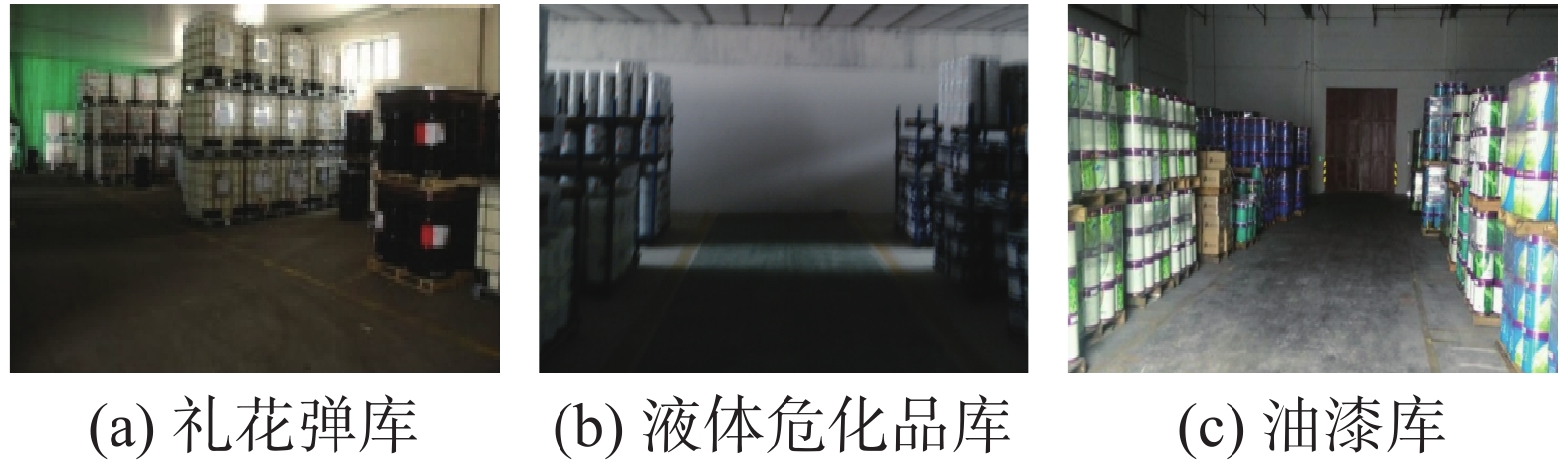Download: 图 4 复杂危化品仓库实景图 Fig. 4 Photos of complex chemical warehouses表 3 Loss统计结果 Tab.3 Statistical loss results表 4 测试结果 Tab.4 Statistical test results

3.4 网络架构适应性测试表 5 适应性测试 Tab.5 Adaptive test results

3.5 细节性实验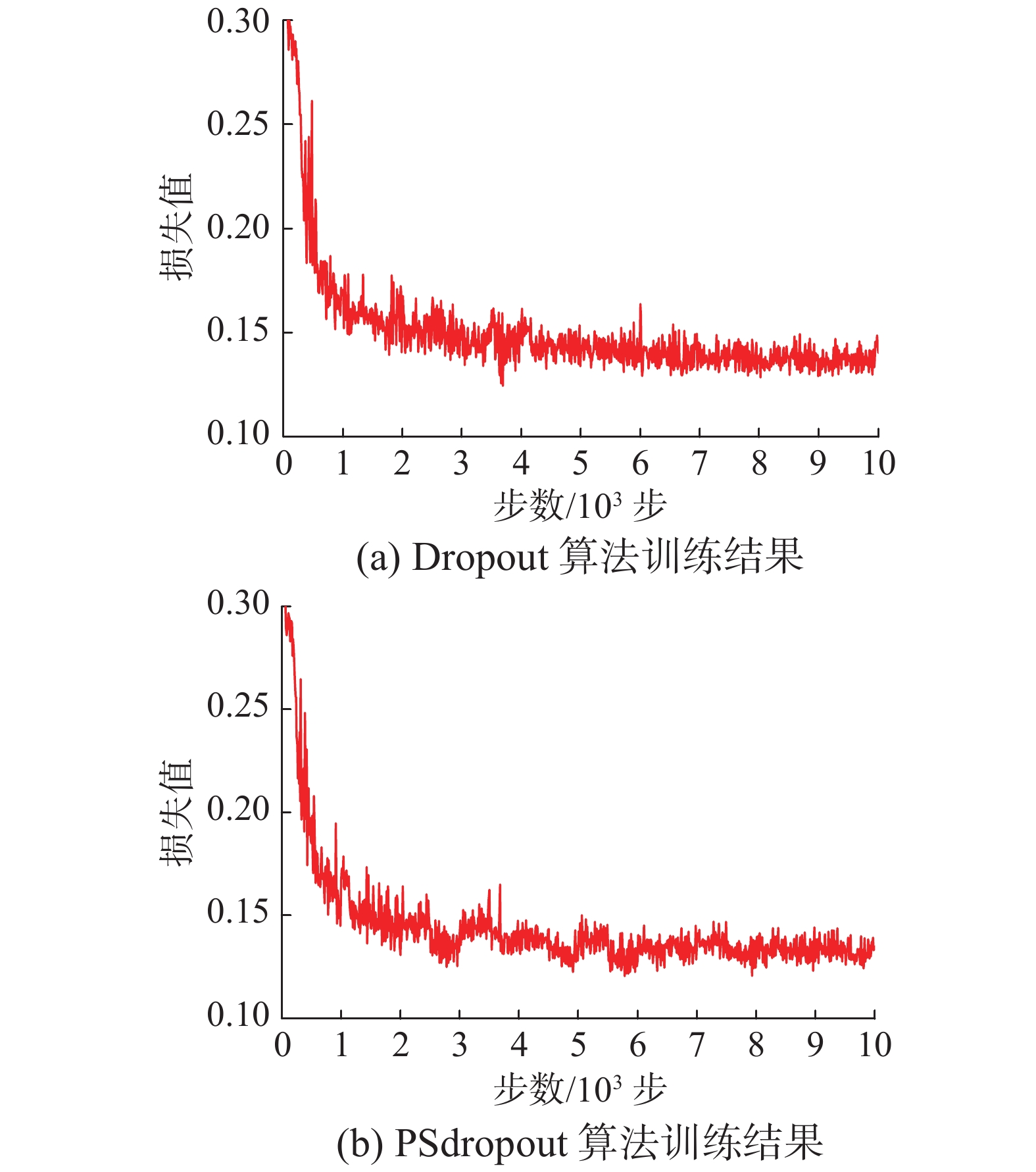Download: 图 5 原始场景数据集上loss统计 Fig. 5 Loss statistics for the original scene data set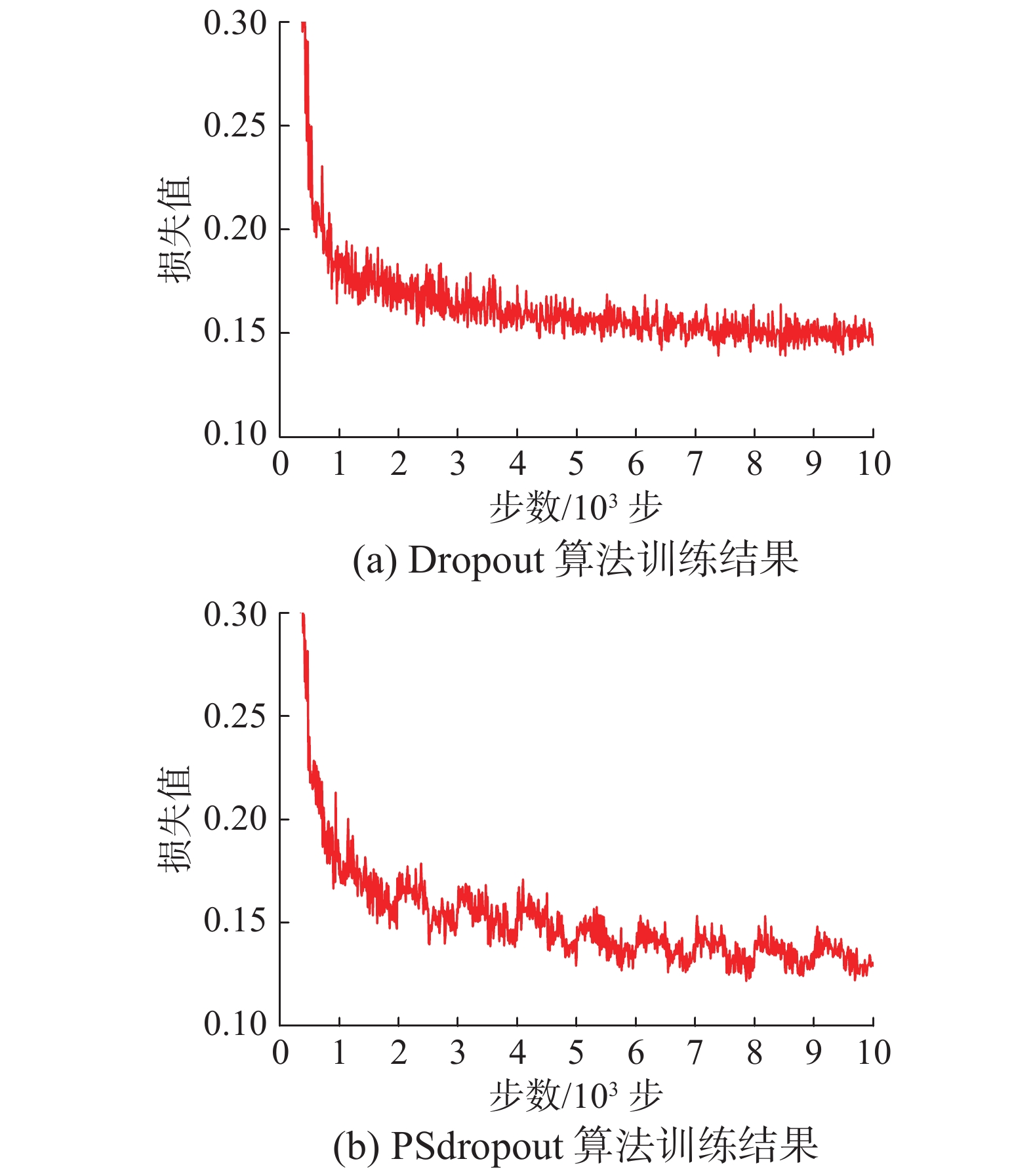Download: 图 6 复杂场景数据集上loss统计 Fig. 6 Loss statistics for the complex scene data set表 6 loss及准确率统计结果 Tab.6 Loss and accuracy statistics

3.6 算法有效性验证实验

Mnist数据集为分类实验公共数据集，在训练网络架构中主要提取的特征为手写体数字的边缘轮廓信息，而本实验提取的信息主要为危化品仓库道路样本的特征信息，侧重点同样在于道路边缘，因此，为了测试PSdropout抽样的稳定性与有效性，采用深度学习经典公共数据集Mnist进行实验，训练集6000张图片，测试集为1000张照片，大小为28×28的灰度照片。

Mnist1：Mnist数据集，学习率调整形式为自动指数衰减，改进函数统计前1000步的训练结果，后续5000步按统计结果更新。

Mnist2：Mnist数据集，学习率为固定值，改进函数统计前1000步的训练结果，后续9000步按统计结果更新。表 7 Mnist数据集上训练结果 Tab.7 Training results for Mnist data set

4 结束语

  刘学君, 江帆, 戴波, 等. 基于ARM的危化品仓库堆垛安全距离监测装置的研究与开发[J]. 制造业自动化, 2016, 38(4): 11-14, 25. LIU Xuejun, JIANG Fan, DAI Bo, et al. The research and development of stack safe distance monitoring device for the chemicals warehouse based on ARM[J]. Manufacturing automation, 2016, 38(4): 11-14, 25. DOI:10.3969/j.issn.1009-0134.2016.04.004 (0)  李琳. 基于UWB定位的危化品仓库管理的设计和研究[D]. 北京: 北京邮电大学, 2018. LI Lin. Design and research of hazardous chemicals warehouse management based on UWB positioning[D]. Beijing: Beijing University of Posts and Telecommunications, 2018. (0)  戴波, 周泽彧, 张岩, 等. 危化品仓储堆垛安全距离监测系统设计[J]. 化工学报, 2019, 70(002): 707-715. DAI Bo, ZHOU Zeyu, ZHANG Yan, et al. Design of safe distance monitoring system for hazardous chemicals storage stack[J]. CIESC Journal, 2019, 70(002): 707-715. (0)  戴波, 吕昕, 刘学君, 等. 基于UWB四参考点矢量补偿的危化品仓储堆垛货物定位方法[J]. 化工学报, 2016, 67(3): 871-877. DAI Bo, LÜ Xin, LIU Xuejun, et al. A UWB-based four reference vectors compensation method applied on hazardous chemicals warehouse stacking positioning[J]. CIESC journal, 2016, 67(3): 871-877. (0)  DAI Bo, LI Yanfei, REN Haisheng, et al. Research on optimization for safe layout of hazardous chemicals warehouse based on genetic algorithm[J]. IFAC-PapersOnline, 2008, 51(18): 245-250. (0)  SRINIVAS S, BABU R V. Data-free parameter pruning for deep neural networks[C]//Proceedings of British Machine Vision Conference 2015. Swansea, UK, 2015: 1−12. (0)  HAN Song, POOL J, TRAN J, et al. Learning both weights and connections for efficient neural networks[C]//Proceedings of the 28th International Conference on Neural Information Processing Systems. Montreal, Quebec, Canada, 2015: 1135−1143. (0)  CHEN Wenlin, WILSON J, TYREE S, et al. Compressing neural networks with the hashing trick[C]//Proceedings of the 32nd International Conference on Machine Learning. Lille, France, 2015: 2285−2294. (0)  HAN Song, MAO Huizi, DALLY W J. Deep compression: compressing deep neural networks with pruning, trained quantization and Huffman coding[C]//Proceedings of 2016 International Conference on Learning Representations. San Juan, Pucrto Rico, 2015: 3−7. (0)  MA Rongrong, NIU Lingfeng. A survey of sparse-learning methods for deep neural networks[C]//Proceedings of 2018 IEEE/WIC/ACM International Conference on Web Intelligence. Santiago, Chile, 2018: 647−650. (0)  李江昀, 赵义凯, 薛卓尔, 等. 深度神经网络模型压缩综述[J]. 工程科学学报, 2019, 41(10): 1229-1239. LI Jiangyun, ZHAO Yikai, XUE Zhuoer, et al. A survey of model compression for deep neural networks[J]. Chinese journal of engineering, 2019, 41(10): 1229-1239. (0)  纪荣嵘, 林绍辉, 晁飞, 等. 深度神经网络压缩与加速综述[J]. 计算机研究与发展, 2018, 55(9): 1871-1888. JI Rongrong, LIN Shaohui, CHAO Fei, et al. Deep neural network compression and acceleration: a review[J]. Journal of computer research and development, 2018, 55(9): 1871-1888. DOI:10.7544/issn1000-1239.2018.20180129 (0)  王晓华, 杨新艳, 焦李成. 基于多尺度几何分析的复杂网络压缩策略[J]. 电子与信息学报, 2009, 31(4): 968-972. WANG Xiaohua, YANG Xinyan, JIAO Licheng. Compression of complex networks based on multiscale geometric analysis[J]. Journal of electronics & information technology, 2009, 31(4): 968-972. (0)  YU Jie, TIAN Shen. A review of network compression based on deep network pruning[C]//Proceedings of the 3rd International Conference on Mechatronics Engineering and Information Technology. Dalian, China, 2019: 324−335. (0)  HINTON G E, SRIVASTAVA N, KRIZHEVSKY A, et al. Improving neural networks by preventing co-adaptation of feature detectors[J]. Computer Science, 2012, 3(4): 212−223. (0)  BALDI P, SADOWSKI P. The dropout learning algorithm[J]. Artificial intelligence, 2014, 210: 78-122. DOI:10.1016/j.artint.2014.02.004 (0)  周飞燕, 金林鹏, 董军. 卷积神经网络研究综述[J]. 计算机学报, 2017, 40(6): 1229-1251. ZHOU Feiyan, JIN Linpeng, DONG Jun. Review of convolutional neural network[J]. Chinese journal of computers, 2017, 40(6): 1229-1251. DOI:10.11897/SP.J.1016.2017.01229 (0)  李彦冬, 郝宗波, 雷航. 卷积神经网络研究综述[J]. 计算机应用, 2016, 36(9): 2508-2515, 2565. LI Yandong, HAO Zongbo, LEI Hang. Survey of convolutional neural network[J]. Journal of computer applications, 2016, 36(9): 2508-2515, 2565. DOI:10.11772/j.issn.1001-9081.2016.09.2508 (0)  陈先昌. 基于卷积神经网络的深度学习算法与应用研究[D]. 杭州: 浙江工商大学, 2014. CHEN Xianchang. Research on algorithm and application of deep learning based on convolutional neural network[D]. Hangzhou: Zhejiang Gongshang University, 2014. (0)  GIRSHICK R, DONAHUE J, DARRELL T, et al. Rich feature hierarchies for accurate object detection and semantic segmentation[C]//Proceedings of 2014 IEEE Conference on Computer Vision and Pattern Recognition. Columbus, USA, 2014: 580−587. (0)  刘亦芃. 一种关于深度学习网络结构的改进策略[D]. 长春: 吉林大学, 2019. LIU Yipeng. A strategy for improving the structure of deep learning network[D]. Changchun: Jilin University, 2019. (0)  杨国亮, 许楠, 李放, 等. 关于非线性激活函数的深度学习分类方法研究[J]. 江西理工大学学报, 2018, 39(3): 76-83. YANG Guoliang, XU Nan, LI Fang, et al. Research on deep learning classification for nonlinear activation function[J]. Journal of Jiangxi University of Science and Technology, 2018, 39(3): 76-83. (0)  张永雄, 王亮明, 李东. 基于多示例深度学习与损失函数优化的交通标志识别算法[J]. 现代电子技术, 2018, 41(15): 133-136, 140. ZHANG Yongxiong, WANG Liangming, LI Dong. Traffic sign recognition algorithm based on multi-instance deep learning and loss function optimization[J]. Modern electronics technique, 2018, 41(15): 133-136, 140. (0)  WAN Li, ZEILER M, ZHANG Sixin, et al. Regularization of neural networks using dropconnect[C]//Proceedings of the 30th International Conference on Machine Learning. Atlanta, GA, USA, 2013: 1058−1066. (0)  徐玉华, 曾明. 泊松分布性质及应用研究[J]. 长江大学学报(自科版), 2006, 04: 132-133. XU Yuhua, ZENG Ming. Poisson distribution properties and application research[J]. Journal of Yangtze University (Natural Science Edition), 2006, 04: 132-133. (0)